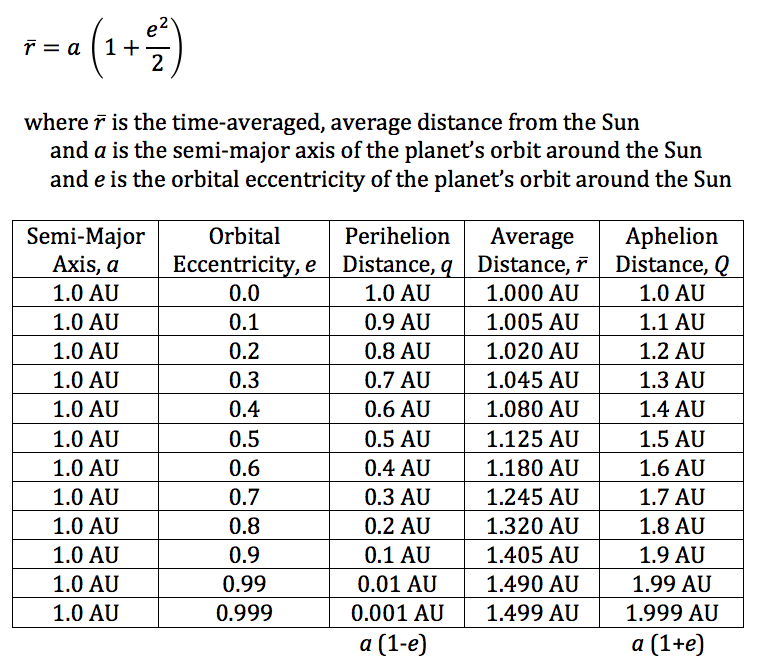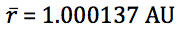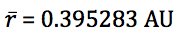## Mercury, Our Nearest Planetary Neighbor

If you’re an astronomy teacher that likes to put a trick question on an open book quiz or test once in a while to encourage your students to think more deeply, here’s a good one for you:

On average, what planet is closest to the Earth?

A. Mars

B. Venus

C. Mercury

The correct answer is C. Mercury.

Huh? Venus comes closest to the Earth, doesn’t it? Yes, but there is a big difference between minimum distance and average distance. Let’s do some quick calculations to help us understand minimum distance first, and then we’ll discuss the more involved determination of average distance.

Here’s some easily-found data on the terrestrial planets:

 Planet a e q Q Mercury 0.387 0.206 Venus 0.723 0.007 Earth 1.000 0.017 Mars 1.524 0.093

I’ve intentionally left the last two columns of the table empty. We’ll come back to those in a moment. a is the semi-major axis of each planet’s orbit around the Sun, in astronomical units (AU). It is often taken that this is the planet’s average distance from the Sun, but that is strictly true only for a circular orbit.1 e is the orbital eccentricity, which is a unitless number. The closer the value is to 0.0, the more circular the orbit. The closer the value is to 1.0, the more elliptical the orbit, with 1.0 being a parabola.

The two empty columns are for q the perihelion distance, and Q the aphelion distance. Perihelion occurs when the planet is closest to the Sun. Aphelion occurs when the planet is farthest from the Sun. How do we calculate the perihelion and aphelion distance? It’s easy.

Perihelion: q = a (1 – e)

Aphelion: q = a (1 + e)

Now, let’s fill in the rest of our table.

 Planet a (AU) e q (AU) Q (AU) Mercury 0.387 0.206 0.307 0.467 Venus 0.723 0.007 0.718 0.728 Earth 1.000 0.017 0.983 1.017 Mars 1.524 0.093 1.382 1.666

Ignoring, for a moment, each planet’s orbital eccentricity, we can calculate the “average” closest approach distance between any two planets by simply taking the difference in their semi-major axes. For Venus, it is 1.000 – 0.723 = 0.277 AU, and for Mars, it is 1.524 – 1.000 = 0.524 AU. We see that Venus comes closest to the Earth.

But, sometimes, Venus and Mars come even closer to the Earth than 0.277 AU and 0.524 AU, respectively. The minimum minimum distance between Venus and the Earth in conjunction should occur when Venus is at aphelion at the same time as Earth is at perihelion: 0.983 – 0.728 = 0.255 AU. The minimum minimum distance between Earth and Mars at opposition should occur when Mars is at perihelion and Earth is at aphelion: 1.382 – 1.017 = 0.365 AU. Mars does not ever come as close to the Earth as Venus does at every close approach.

The above assumes that all the terrestrial planets orbit in the same plane, which they do not. Mercury has an orbital inclination relative to the ecliptic of 7.004˚, Venus 3.395˚, Earth 0.000˚ (by definition), and Mars 1.848˚. Calculating the distances in 3D will change the values a little, but not by much.

Now let’s switch gears and find the average distance over time between Earth and the other terrestrial planets—a very different question. But we want to pick a time period to average over that is sufficiently long enough that each planet spends as much time on the opposite side of the Sun from us as it does on our side of the Sun. The time interval between successive conjunctions (in the case of Mercury and Venus) or oppositions (Mars) is called the synodic period and is calculated as follows:

P1 = 87.9691d = orbital period of Mercury

P2 = 224.701d = orbital period of Venus

P3 = 365.256d = orbital period of Earth

P4 = 686.971d = orbital period of Mars

S1 = (P1-1 – P3-1)-1 = synodic period of Mercury = 115.877d

S2 = (P2-1 – P3-1)-1 = synodic period of Venus = 583.924d

S4 = (P3-1 – P4-1)-1 = synodic period of Mars = 779.946d

I wrote a quick little SAS program to numerically determine that an interval of 9,387 days (25.7 years) would be a good choice, because

9387 / 115.877 = 81.0083, for Mercury

9387 / 583.924 = 16.0757, for Venus

9387 / 779.946 = 12.0354, for Mars

The U.S Naval Observatory provides a free computer program called the Multiyear Interactive Computer Almanac (MICA), so I was able to quickly generate a file for each planet, Mercury, Venus, and Mars, giving the Earth-to-planet distance for 9,387 days beginning 0h UT 1 May 2019 through 0h UT 10 Jan 2045. Here are the results:

 Planet Mean (AU) Median (AU) Min (AU) Max (AU) Mercury 1.039022 1.073148 0.549144 1.451501 Venus 1.138383 1.238453 0.265260 1.735280 Mars 1.711176 1.842260 0.380420 2.675330

As you can see, averaged over time, Mercury is the nearest planet to the Earth!

For a more mathematical treatment, see the article in the 12 Mar 2019 issue of Physics Today.

1 See my article Average Orbital Distance for details.

## Average Orbital Distance

If a planet is orbiting the Sun with a semi-major axis, a, and orbital eccentricity, e, it is often stated that the average distance of the planet from the Sun is simply a.  This is only true for circular orbits (e = 0) where the planet maintains a constant distance from the Sun, and that distance is a.

Let’s imagine a hypothetical planet much like the Earth that has a perfectly circular orbit around the Sun with a = 1.0 AU and e = 0.  It is easy to see in this case that at all times, the planet will be exactly 1.0 AU from the Sun.

If, however, the planet orbits the Sun in an elliptical orbit at a = 1 AU and e > 0, we find that the planet orbits more slowly when it is farther from Sun than when it is nearer the Sun.  So, you’d expect to see the time-averaged average distance to be greater than 1.0 AU.  This is indeed the case.The Earth’s current osculating orbital elements give us:

a = 0.999998 and e = 0.016694

Earth’s average distance from the Sun is thus:Mercury, the innermost planet, has the most eccentric orbit of all the major planets:

a = 0.387098 and e = 0.205638

Mercury’s average distance from the Sun is thus: